SBOS122E December   1999  – December 2017

PRODUCTION DATA.

1. Features
2. Applications
3. Description
4. Revision History
5. Pin Configuration and Functions
6. Specifications
7. Detailed Description
1. 7.1 Overview
2. 7.2 Functional Block Diagram
3. 7.3 Feature Description
4. 7.4 Device Functional Modes
8. Application and Implementation
1. 8.1 Application Information
2. 8.2 Typical Applications
1. 8.2.1 Buffering Output to Drive an ADC
2. 8.2.2 Output Filter
3. 8.2.3 Offsetting the Output Voltage
4. 8.2.4 Bipolar Current Measurement
5. 8.2.5 Bipolar Current Measurement Using Differential Input of ADC
6. 8.2.6 Multiplexed Measurement Using Logic Signal for Power
9. Power Supply Recommendations
10. 10Layout
11. 11Device and Documentation Support
12. 12Mechanical, Packaging, and Orderable Information

• DBV|5

## 8 Application and Implementation

NOTE

Information in the following applications sections is not part of the TI component specification, and TI does not warrant its accuracy or completeness. TI’s customers are responsible for determining suitability of components for their purposes. Customers should validate and test their design implementation to confirm system functionality.

### 8.1 Application Information

#### 8.1.1 Operation

Figure 9 illustrates the basic circuit diagram for both the INA138 and INA168 devices. Load current IS is drawn from supply VS through shunt resistor RS. The voltage drop in shunt resistor VS is forced across RG1 by the internal op amp, causing current to flow into the collector of Q1. External resistor RL converts the output current to a voltage, VOUT, at the OUT pin. The transfer function for the INA138 device is:

Equation 3. IO = gm(VIN+ – VIN–)

where gm = 200 µA/V.

In the circuit of Figure 9, the input voltage, (VIN+ – VIN–), is equal to IS × RS and the output voltage, VOUT, is equal to IO × RL. The transconductance, gm, of the INA138 device is 200 µA/V. The complete transfer function for the current measurement amplifier in this application is:

Equation 4. VOUT = (IS) (RS) (200 µA/V) (RL)

The maximum differential input voltage for accurate measurements is 0.5 V, which produces a 100-µA output current. A differential input voltage of up to 2 V will not cause damage. Differential measurements (pins 3 and 4) must be unipolar with a more-positive voltage applied to pin 3. If a more-negative voltage is applied to pin 3, the output current, IO, will be zero, but it will not cause damage.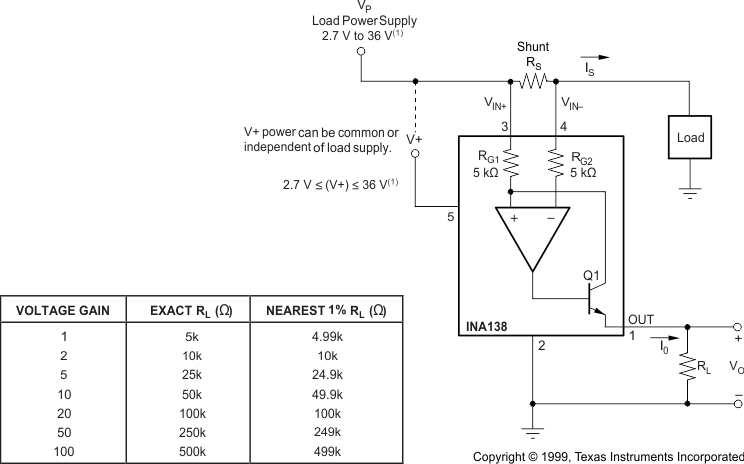Maximum VP and V+ voltage is 60 V with INA168.
Figure 9. Basic Circuit Connections

### 8.2 Typical Applications

The INA1x8 devices are designed for current shunt measurement circuits, as shown in Figure 9, but basic device function is useful in a wide range of circuitry. A creative engineer will find many unforeseen uses in measurement and level-shifting circuits. A few ideas are illustrated in Figure 10 through Figure 18.

#### 8.2.1 Buffering Output to Drive an ADC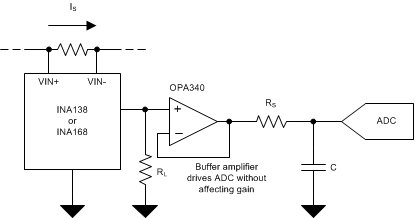Figure 10. Buffering Output to Drive an ADC

#### 8.2.1.1 Design Requirements

Digitize the output of the INA1x8 devices using a 1-MSPS analog-to-digital converter (ADC).

#### 8.2.1.2.1 Selecting the Shunt Resistor and RL

In Figure 9 the value chosen for the shunt resistor depends on the application and is a compromise between small-signal accuracy and maximum permissible voltage loss in the measurement line. High values of shunt resistor provide better accuracy at lower currents by minimizing the effects of offset, while low values of shunt resistor minimize voltage loss in the supply line. For most applications, best performance is attained with a shunt resistor value that provides a full-scale shunt voltage range of 50 mV to 100 mV. Maximum input voltage for accurate measurements is 500 mV.

The load resistor, RL, is chosen to provide the desired full-scale output voltage. The output impedance of the INA1x8 OUT terminal is very high which permits using values of RL up to 500 kΩ with excellent accuracy. The input impedance of any additional circuitry at the output should be much higher than the value of RL to avoid degrading accuracy.

Some analog-to-digital converters (ADCs) have input impedances that significantly affect measurement gain. The input impedance of the ADC can be included as part of the effective RL if its input can be modeled as a resistor to ground. Alternatively, an op amp can be used to buffer the ADC input. The INA1x8 are current output devices, and as such have an inherently large output impedance. The output currents from the amplifier are converted to an output voltage via the load resistor, RL, connected from the amplifier output to ground. The ratio of the load resistor value to that of the internal resistor value determines the voltage gain of the system.

In many applications digitizing the output of the INA1x8 device is required, and can be accomplished by connecting the output of the amplifier to an ADC. It is very common for an ADC to have a dynamic input impedance. If the INA1x8 output is connected directly to an ADC input, the input impedance of the ADC is effectively connected in parallel with the gain setting resistor RL. This parallel impedance combination will affect the gain of the system and the impact on the gain is difficult to estimate accurately. A simple solution that eliminates the paralleling of impedances, simplifying the gain of the circuit is to place a buffer amplifier, such as the OPA340, between the output of the INA138 or INA168 device and the input to the ADC.

Figure 10 illustrates this concept. Notice that a low pass filter is placed between the OPA340 output and the input to the ADC. The filter capacitor is required to provide any instantaneous demand for current required by the input stage of the ADC. The filter resistor is required to isolate the OPA340 output from the filter capacitor to maintain circuit stability. The values for the filter components will vary according to the operational amplifier used for the buffer and the particular ADC selected. More information can be found regarding the design of the low pass filter in the TI Precision Design , 16 bit 1MSPS Data Acquisition Reference Design for Single-Ended Multiplexed Applications (TIPD173).

Figure 11 shows the expected results when driving an analog-to-digital converter at 1MSPS with and without buffering the INA1x8 output. Without the buffer, the high impedance of the INA1x8 reacts with the input capacitance and sample and hold (S/H) capacitance of the ADC, and does not allow the S/H to reach the correct final value before it is reset and the next conversion starts. Adding the buffer amplifier significantly reduces the output impedance driving the S/H and allows for higher conversion rates than can be achieved without adding the buffer.

#### 8.2.1.3 Application Curve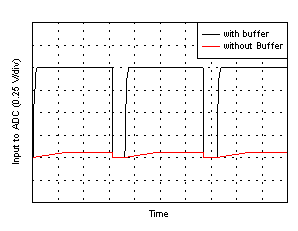Figure 11. Driving an ADC With and Without a Buffer

#### 8.2.2 Output Filter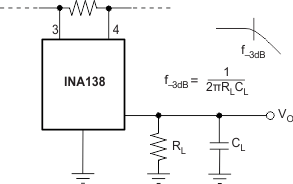Figure 12. Output Filter

#### 8.2.2.1 Design Requirements

Filter the output of the INA1x8 devices.

#### 8.2.2.2 Detailed Design Procedure

A low-pass filter can be formed at the output of the INA1x8 devices simply by placing a capacitor of the desired value in parallel with the load resistor. First determine the value of the load resistor needed to achieve the desired gain. Refer to the table in Figure 9. Next, determine the capacitor value that will result in the desired cutoff frequency according to the equation shown in Figure 12. Figure 13 illustrates various combinations of gain settings (determined by RL) and filter capacitors.

#### 8.2.2.3 Application Curve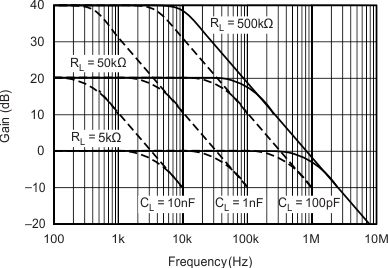Figure 13. Gain vs Frequency

#### 8.2.3 Offsetting the Output Voltage

For many applications using only a single power supply it may be required to level shift the output voltage away from ground when there is no load current flowing in the shunt resistor. Level shifting the output of the INA1x8 devices is easily accomplished by one of two simple methods shown in Figure 14. The method on the left hand side of Figure 14 illustrates a simple voltage divider method. This method is useful for applications that require the output of the INA1x8 devices to remain centered with respect to the power supply at zero load current through the shunt resistor. Using this method the gain is determine by the parallel combination of R1 and R2 while the output offset is determined by the voltage divider ratio R1 and R2. For applications that may require a fixed value of output offset, independent of the power supply voltage, the current source method shown on the right-hand side of Figure 14 is recommended. With this method a REF200 constant current source is used to generate a constant output offset. Using his method the gain is determined by RL and the offset is determined by the product of the value of the current source and RL.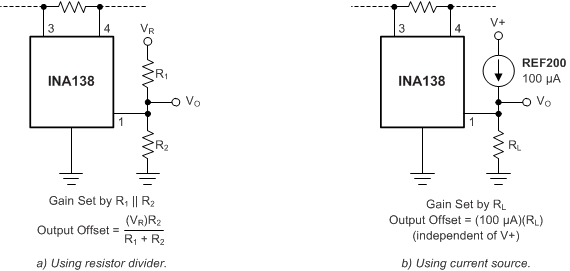Figure 14. Offsetting the Output Voltage

#### 8.2.4 Bipolar Current Measurement

The INA1x8 devices can be configured as shown in Figure 15 in applications where measuring current bi-directionally is required. Two INA devices are required connecting their inputs across the shunt resistor as shown in Figure 15. A comparator, such as the TLV3201, is used to detect the polarity of the load current. The magnitude of the load current is monitored across the resistor connected between ground and the connection labeled Output. In this example the 100-kΩ resistor results in a gain of 20 V/V. The 10-kΩ resistors connected in series with the INA1x8 output current are used to develop a voltage across the comparator inputs. Two diodes are required to prevent current flow into the INA1x8 output, as only one device at a time is providing current to the Output connection of the circuit. The circuit functionality is illustrated in Figure 16.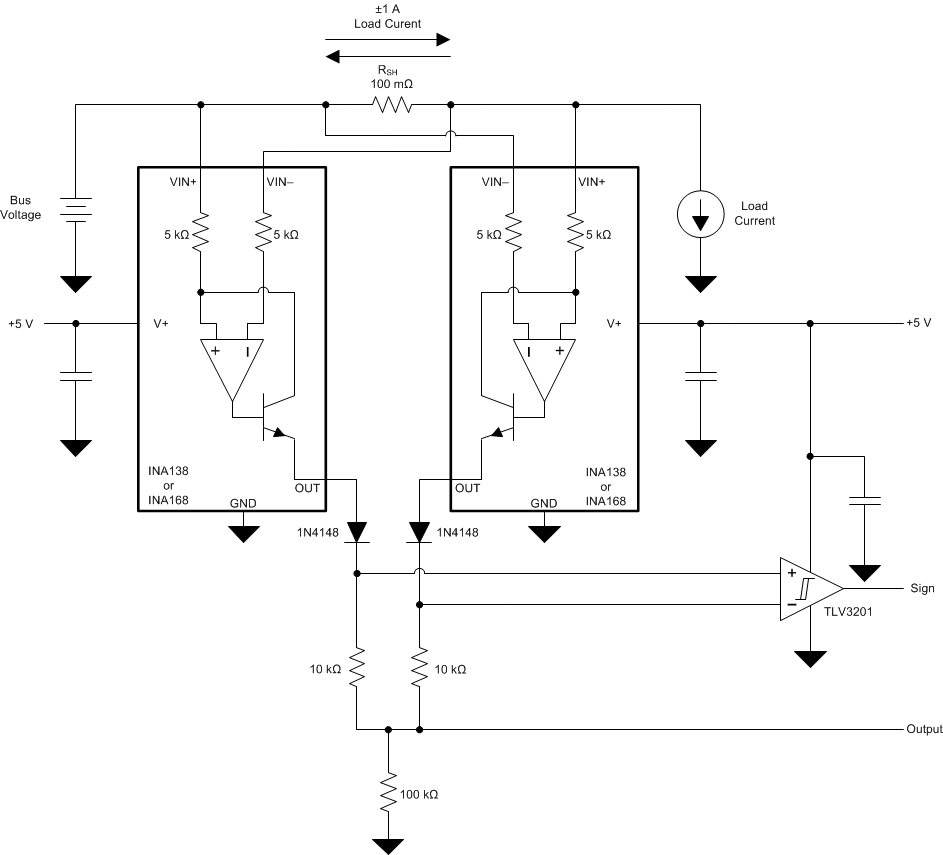Figure 15. Bipolar Current Measurement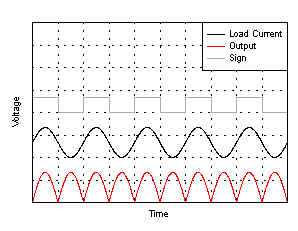Figure 16. Bipolar Current Measurements Results (arbitrary scale)

#### 8.2.5 Bipolar Current Measurement Using Differential Input of ADC

The INA1x8 devices can be used with an ADC such as the ADS7870 programmed for differential mode operation. Figure 17 illustrates this configuration. In this configuration the use of two INAs allows for bidirectional current measurement. Depending upon the polarity of the current, one of the INAs provides an output voltage, while the other output is zero. In this way the ADC reads the polarity of current directly, without the need for additional circuitry.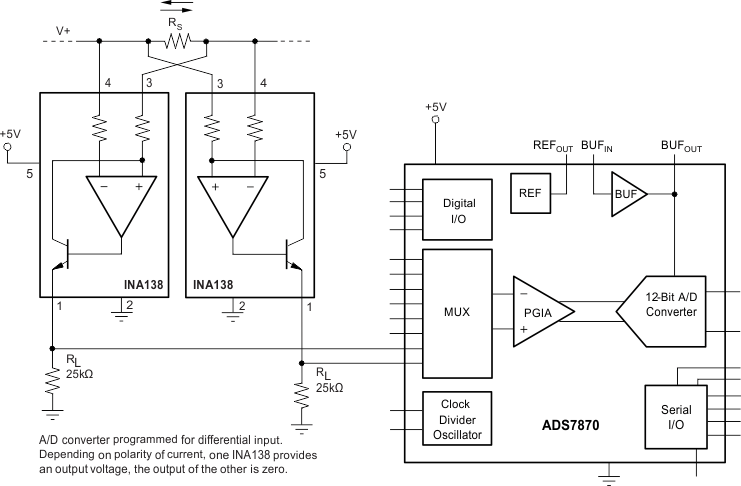Figure 17. Bipolar Current Measurement Using Differential Input of ADC

#### 8.2.6 Multiplexed Measurement Using Logic Signal for Power

Multiple loads can be measured as illustrated in Figure 18. In this configuration each INA1x8 device is powered by the digital I/O from the ADS7870. Multiplexing is achieved by switching on or off each the desired I/O.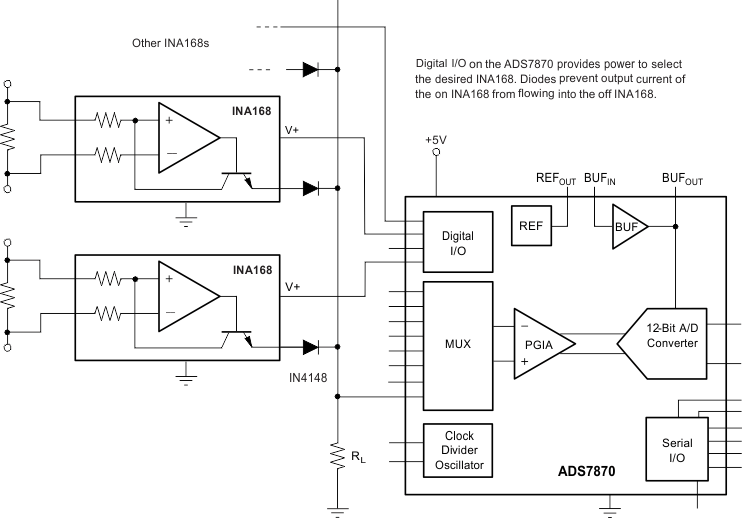Figure 18. Multiplexed Measurement Using Logic Signal for Power